## What's in the Circle?

In a circle puzzle like the ones below, dashed arrows mean to add and solid arrows mean to multiply. For example, the solution to the puzzle on the left is a number whose sum is $5 + 9,$ which is $14.$ The solution to the puzzle on the right is a number that, when multiplied by $6,$ gives us $12.$ By working backwards, we get a solution of $2.$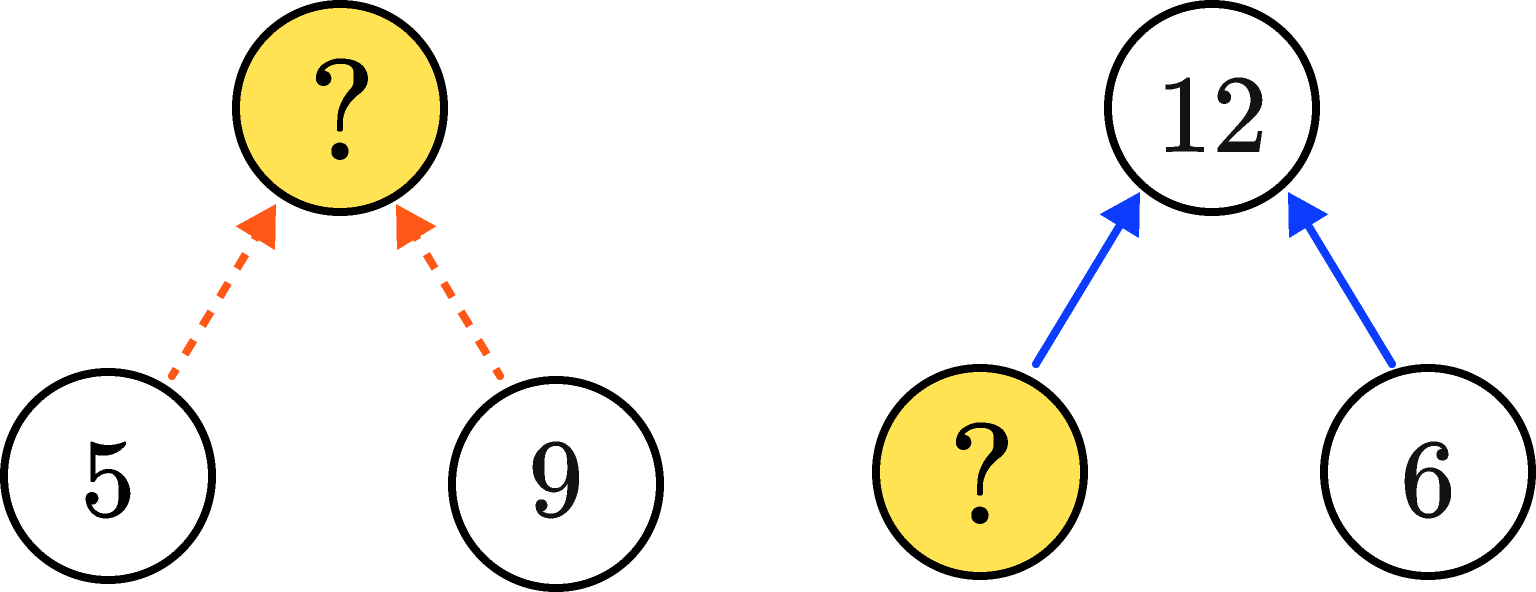Keep reading to see a few more complicated example puzzles, or dive right into today's challenge if you feel ready.

The two circle puzzles we just saw are both one-step puzzles. To solve multi-step circle puzzles, we might need to use multiple arithmetic steps in the correct order.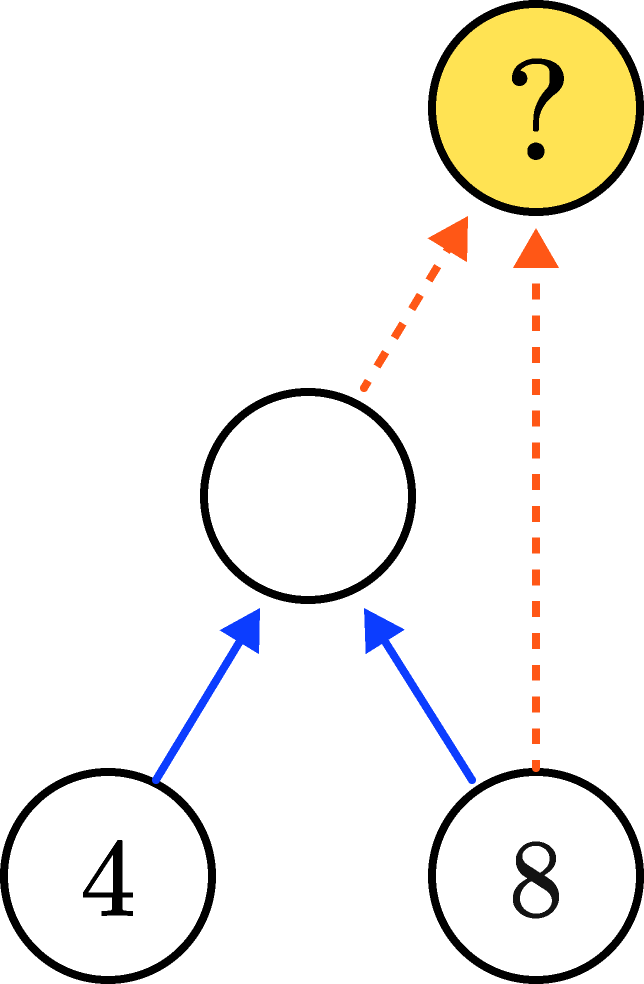The solution number is the sum of $8$ and another unknown number. We can find the unknown number since it's the product of $4$ and $8,$ which is $32.$ The solution must then be $32 + 8 = \mathbf{40}.$

On the other hand, some multi-step puzzles require us to work backwards through multiple steps.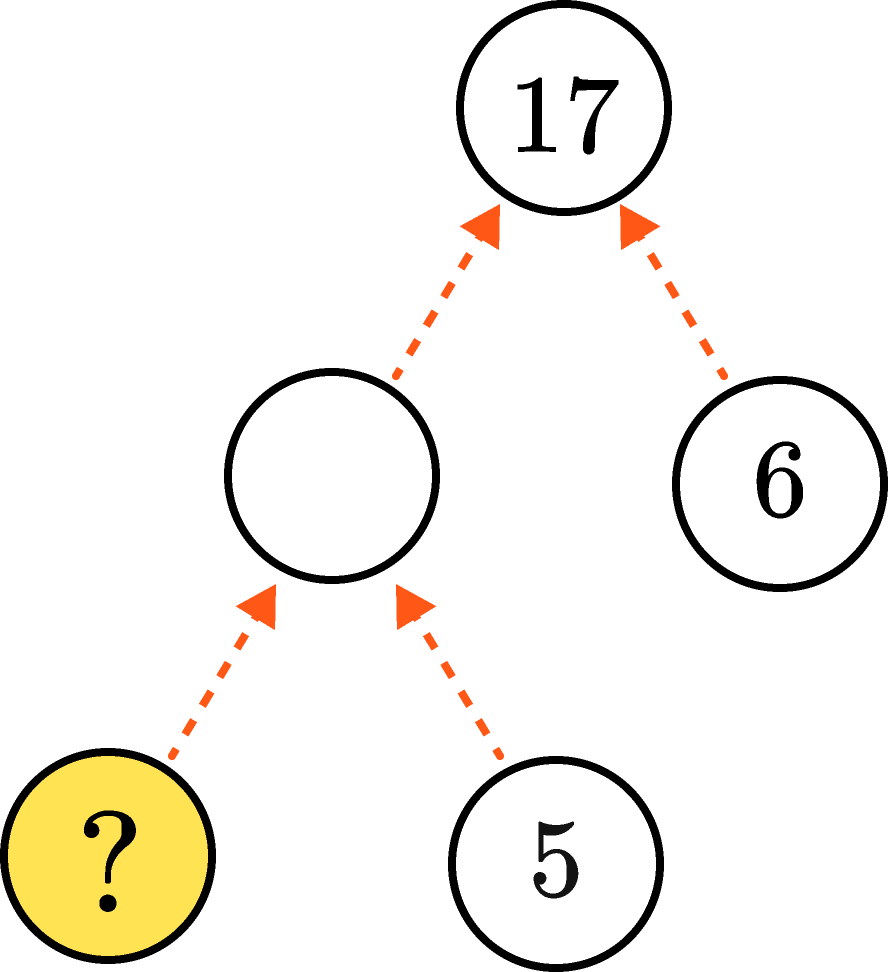Now, the solution number and $5$ add to some unknown number. We need to find that unknown number first. We can do so since it adds to $6$ to give us $17.$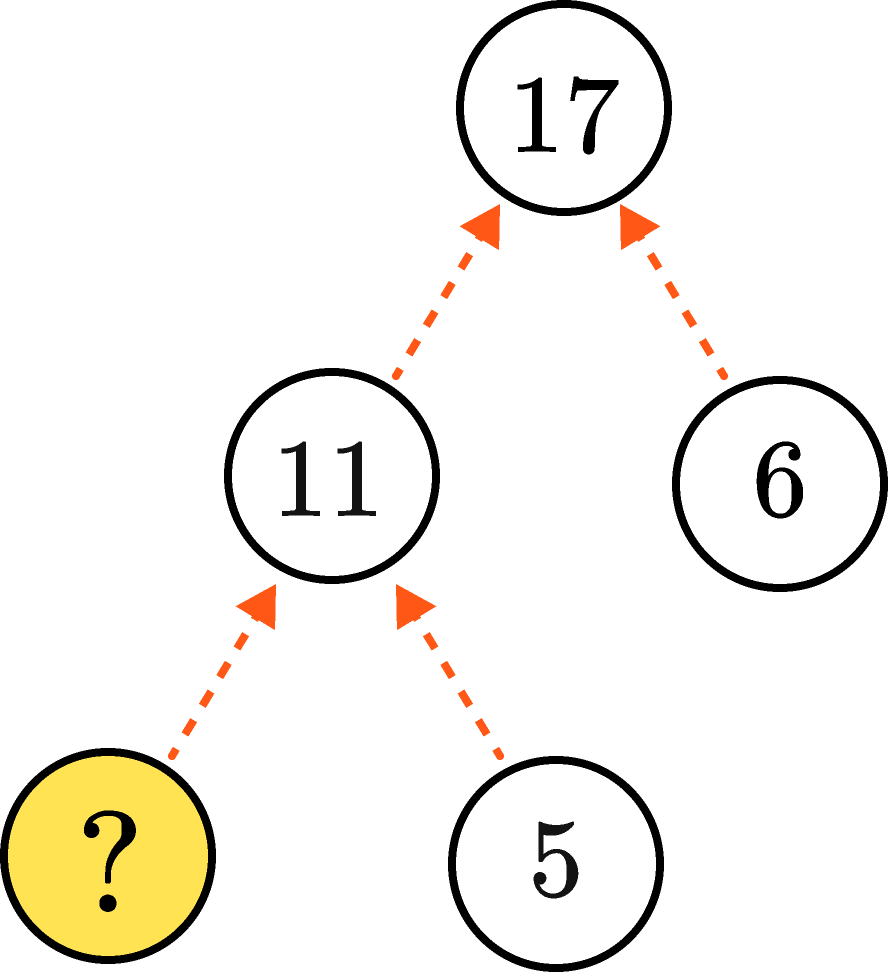The unknown number was $11,$ and it is the sum of our solution number and $5.$ This means our solution is $6.$

We can simplify some complex puzzles to make them easier to solve. In the puzzle below, $48$ is the product of three numbers: $2,$ the solution number, and some other unknown number.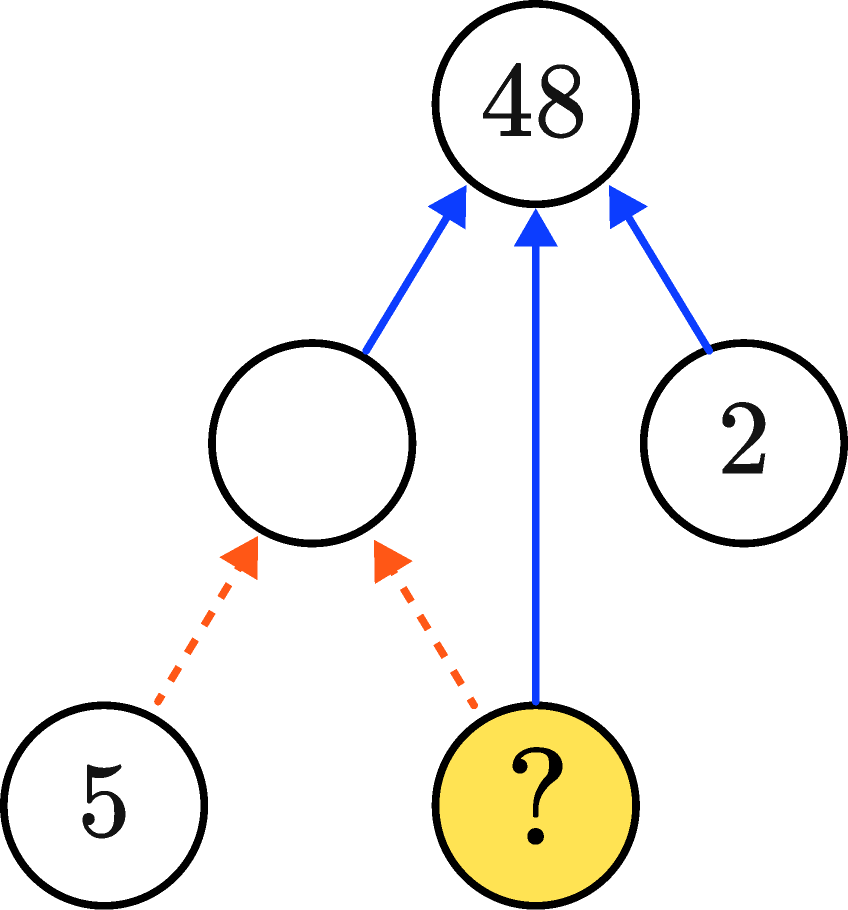Since $2 \times 24 = 48,$ the solution number and the other unknown number must multiply to $24.$ We can then get rid of the $2$ and show $24$ as a product of two numbers.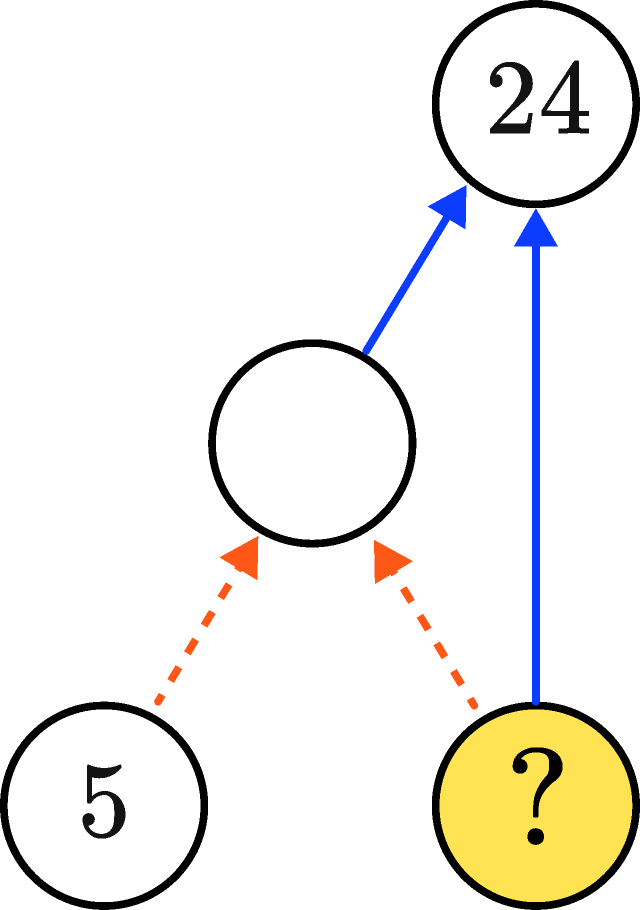The puzzle now tells us that $24$ is the product of two numbers: the solution number, and five added to the solution number. Let's list factor pairs of $24$ to find the solution:

\begin{aligned} 24 & \times 1 &&&&& (-24) \times (-1) \\ 12 & \times 2 &&&&& (-12) \times (-2) \\ 8 & \times 3 &&&&& (-8) \times (-3) \\ 6 & \times 4 &&&&& (-6) \times (-4) \\ \end{aligned}

There are two factor pairs whose numbers have a difference of $5.$ These pairs are $(8, 3)$ and $(-8, -3).$ This means there are two solutions: $3$ and $-8.$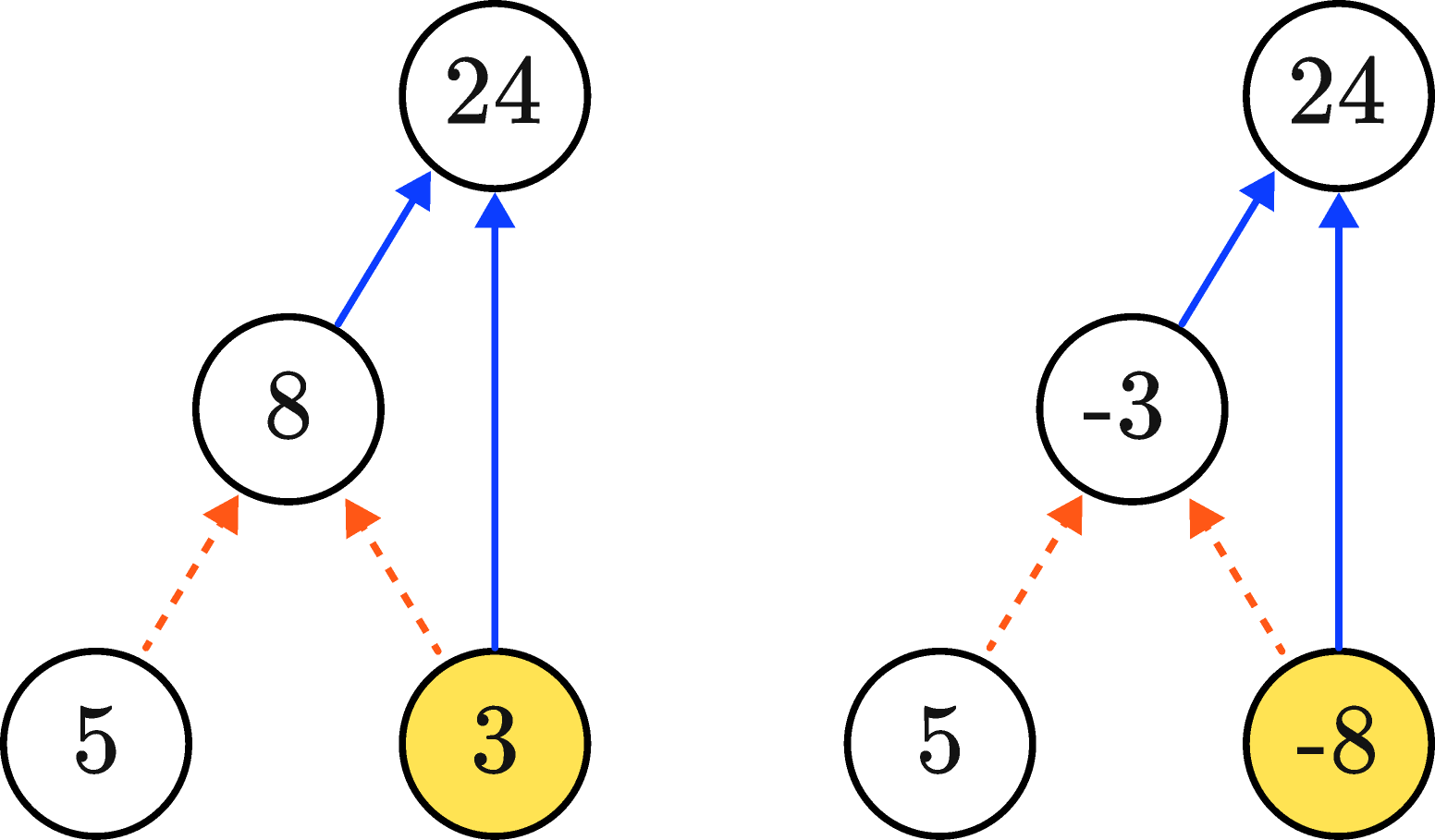Can you solve the circle puzzle below?

# Today's Challenge

Dashed arrows mean addition, while solid arrows mean multiplication. What number goes in the place of the question mark?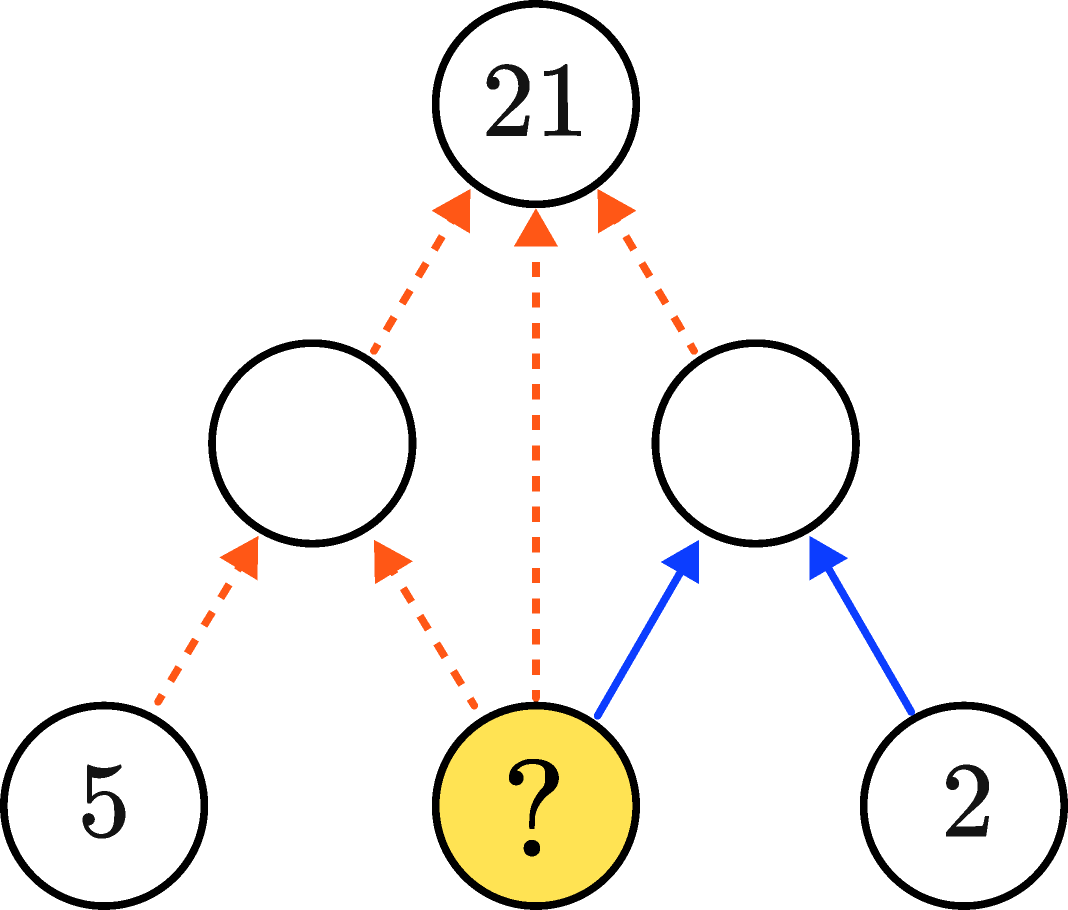×